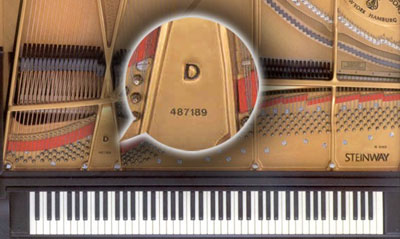# HOW OLD IS MY STEINWAY?

To determine the age of a Steinway piano we need to first locate the serial number.

##On Steinway upright pianos the serial number can be located on top of the wrest plank (pinblock) above the cast iron plate when you open the lid.

##On Steinway grand pianos the serial number can be found on the cast iron plate between the tuning pins above the keyboard.

## Steinway Serial Numbers by Year Manufactured

Please Note: Serial numbers represent the approximate beginning serial number for the year of production for New York and Hamburg pianos.  Steinway Serial numbers for some early production may span more than one year.

 1000 = 1856 100000 = 1901 276000 = 1933 385000 = 1964 531000 = 1995 2000 = 1858 105000 = 1902 278000 = 1934 390000 = 1965 533500 = 1996 3000 = 1860 110000 = 1904 279000 = 1935 395000 = 1966 538000 = 1997 5000 = 1861 115000 = 1905 284000 = 1936 400000 = 1967 541000= 1998 7000 = 1863 120000 = 1906 289000 = 1937 405000 = 1968 546000 = 1999 9000 = 1864 125000 = 1907 290000 = 1938 412000 = 1969 550000 = 2000 11000 = 1865 130000 = 1908 294000 = 1939 418000 = 1970 555000 = 2001 13000 = 1866 135000 = 1909 300000 = 1940 423000 = 1971 559000 = 2002 15000 = 1867 140000 = 1910 305000 = 1941 426000 = 1972 563000 = 2003 17000 = 1868 150000 = 1911 310000 = 1942 431000 = 1973 567000 = 2004 19000 = 1869 155000 = 1912 314000 = 1943 436000 = 1974 571000 = 2005 21000 = 1870 160000 = 1913 316000 = 1944 439000 = 1975 574500 = 2006 23000 = 1871 165000 = 1914 317000 = 1945 445000 = 1976 578000 = 2007 25000 = 1872 170000 = 1915 319000 = 1946 450000 = 1977 582000 = 2008 27000 = 1873 175000 = 1916 322000 = 1947 455500 = 1978 584500 = 2009 29000 = 1874 183000 = 1917 324000 = 1948 463000 = 1979 587000 = 2010 31000 = 1875 190000 = 1918 328000 = 1949 468500 = 1980 589500 = 2011 33000 = 1876 195000 = 1919 331000 = 1950 473500 = 1981 592000 = 2012 35000 = 1877 200000 = 1920 334000 = 1951 478500 = 1982 594500 = 2013 40000 = 1878 205000 = 1921 337000 = 1952 483000 = 1983 597500 = 2014 45000 = 1881 210000 = 1922 340000 = 1953 488000 = 1984 600000 = 2015 50000 = 1883 217000 = 1923 343000 = 1954 493000 = 1985 603000 = 2016 55000 = 1886 225000 = 1924 346500 = 1955 498000 = 1986 606500 = 2017 60000 = 1887 232000 = 1925 350000 = 1956 500000 = 1987 608500 = 2018 65000 = 1889 240000 = 1926 355000 = 1957 504000 = 1988 611500 = 2019 70000 = 1891 249000 = 1927 358000 = 1958 508000 = 1989 614000 = 2020 75000 = 1893 260000 = 1928 362000 = 1959 513000 = 1990 617000 = 2021 80000 = 1894 263000 = 1929 366000 = 1960 517000 = 1991 620000 = 2022 85000 = 1896 269000 = 1930 370000 = 1961 521000 = 1992 90000 = 1898 271000 = 1931 375000 = 1962 524000 = 1993 95000 = 1900 274000 = 1932 380000 = 1963 527000 = 1994

## Important Steinway Patents

Year Patents Accumulated By The Year Patents Missing If You Bought In The Year Select patents
2009 126 13
1999 123 16 2000 Piano Sostenuto Assembly, 2003 Damper Adjustment Device
1989 116 23 1992 Piano Hammer Forming Method and Reinforcement, 1996 Piano Escapement Action
1979 115 24 1983 "Permafree II" Bushing Cloth
1969 114 25 1976 Elastomeric Resin Bushing for Piano Action
1959 110 29 1963 Hexagrip Wrestplank
1949 107 32 1959 Action with Means for Adjusting the Touch of Keys
1939 93 46 1944 Piano Pinblock, 1945 High-Frequency Molding Method
1929 75 64 1936 Accelerated Action, Diaphragmatic Soundboard
1919 74 65 1923 Tracker Board for Player Pianos
1909 72 67 1911 Grand Underlever Top Flange w/Flexible Tab
1899 68 71 1903 Upright Plate--Cast Capo Bar, Inverted Scale Rib, Transverse Braces
1889 61 78 1885 Grand Treble Bell, Grand Double Cupola Plate, Upight Closing Rim
1879 40 99 1872 Grand Duplex Agraffe Scale, 1874 Sostenuto Mechanism
1869 11 128 1868-69 Upright and Grand Tubular Metallic Action Frames
1859 3 136 1859 Grand Overstringing
1853 0 139 1857 Square and Grand Actions